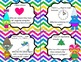# Geometry: I Have..Who Has? SBAC, PARCC Test PrepSubject
Resource Type
File Type

PDF

(58 MB|14 pages)
Product Rating
Standards
• Product Description
• StandardsNEW

This is a 36 card set that includes an answer key and ways to use it in your class. This is not just a game for learning vocabulary. It incorporates critical thinking and reasoning skills.

Students will learn:

right angle

straight angle

acute angle

obtuse angle

reflex angle

angles in relationship to the clock

pentagon

trapezoid

rhombus

square

circle

oval

parallelogram

equilateral triangle

isosceles triangle

scalene triangle

vertex

angle

symmetry

perpendicular lines

parallel lines

center

ray

line segment

line

diagonal

Reason abstractly and quantitatively. Mathematically proficient students make sense of quantities and their relationships in problem situations. They bring two complementary abilities to bear on problems involving quantitative relationships: the ability to decontextualize-to abstract a given situation and represent it symbolically and manipulate the representing symbols as if they have a life of their own, without necessarily attending to their referents-and the ability to contextualize, to pause as needed during the manipulation process in order to probe into the referents for the symbols involved. Quantitative reasoning entails habits of creating a coherent representation of the problem at hand; considering the units involved; attending to the meaning of quantities, not just how to compute them; and knowing and flexibly using different properties of operations and objects.
Recognize a line of symmetry for a two-dimensional figure as a line across the figure such that the figure can be folded along the line into matching parts. Identify line-symmetric figures and draw lines of symmetry.
Classify two-dimensional figures based on the presence or absence of parallel or perpendicular lines, or the presence or absence of angles of a specified size. Recognize right triangles as a category, and identify right triangles.
Recognize angle measure as additive. When an angle is decomposed into non-overlapping parts, the angle measure of the whole is the sum of the angle measures of the parts. Solve addition and subtraction problems to find unknown angles on a diagram in real world and mathematical problems, e.g., by using an equation with a symbol for the unknown angle measure.
An angle is measured with reference to a circle with its center at the common endpoint of the rays, by considering the fraction of the circular arc between the points where the two rays intersect the circle. An angle that turns through 1/360 of a circle is called a “one-degree angle,” and can be used to measure angles.
Total Pages
14 pages
Included
Teaching Duration
N/A
Report this Resource to TpT
Reported resources will be reviewed by our team. Report this resource to let us know if this resource violates TpT’s content guidelines.